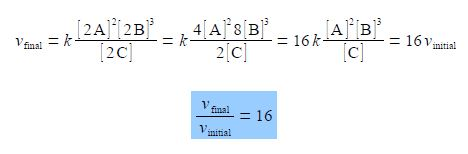# Rates of Chemical Reactions and Concentrations

A + B → C

A particular chemical reactions has a rate or speed v (“vee” as in velocity) given in terms of the concentrations of the reactants and products (indicted by square brackets) and the rate constant k. For clarity we have not included the units in the rate equation, this is the normal convention in kinetic rate equations purely for clarity.

This particular reaction’s rate is experimentally found to depend upon the square of the concentration of the reactant A, the cube of the concentration of the reactant B and is inversely proportional to the concentration of the product C.

vinitial = K {([A]2 [B]3)/ [C]}

Calculate the rate of reaction if the concentrations of A, B, C are all doubled by finding the ratio of the final rate of reaction, vfinal, to the initial rate of reaction vinitial.

Solution

The final rate vfinal is with all the above concentrations doubled and with the powers applying to the new doubled concentrations. The equation is cleaned up by taking the numerical factors outside the brackets.The ratio of the final rate of reaction vfinal to the initial rate of reaction vinitial is 16. Doubling all the concentrations has made the reaction go 16 times faster!